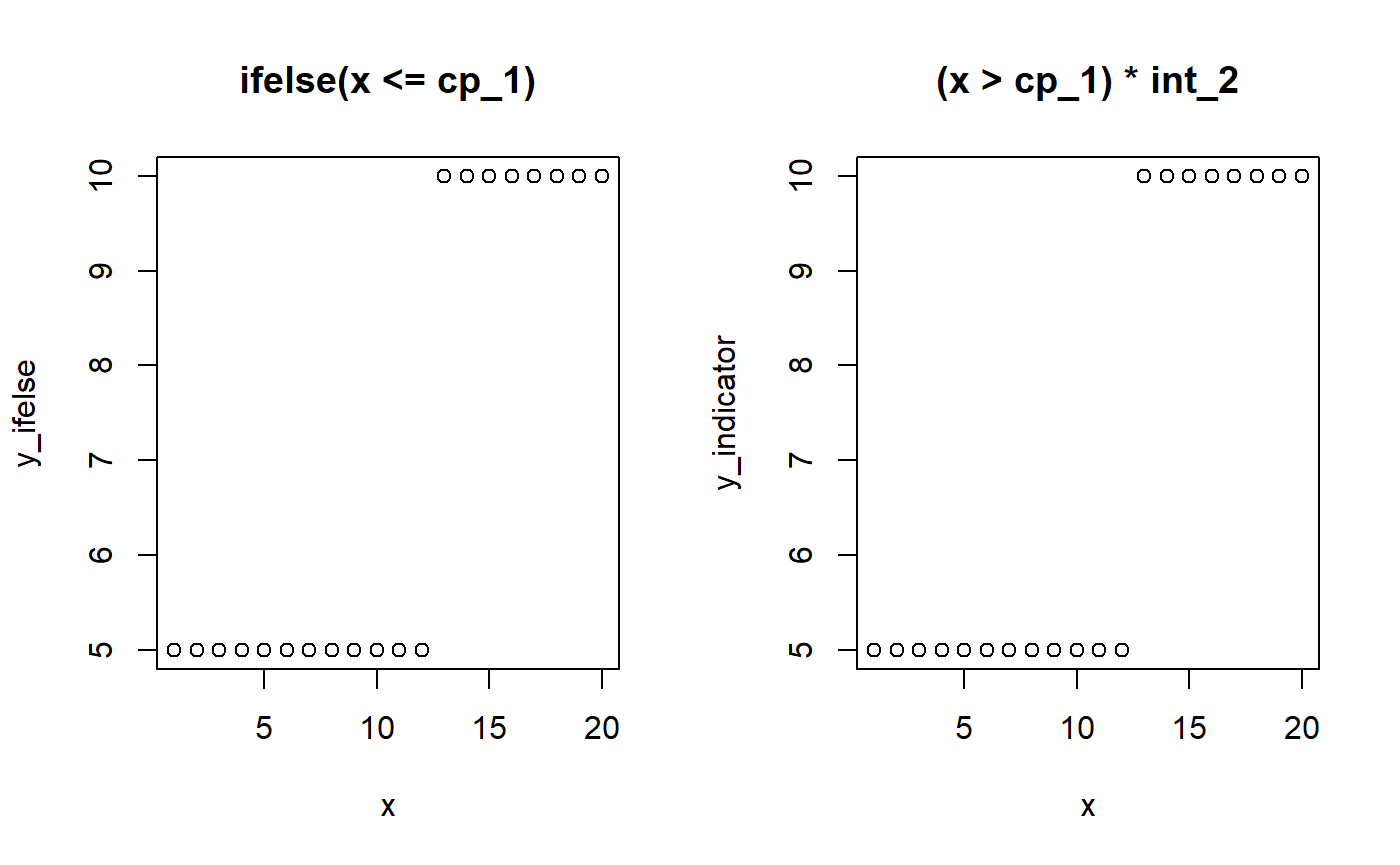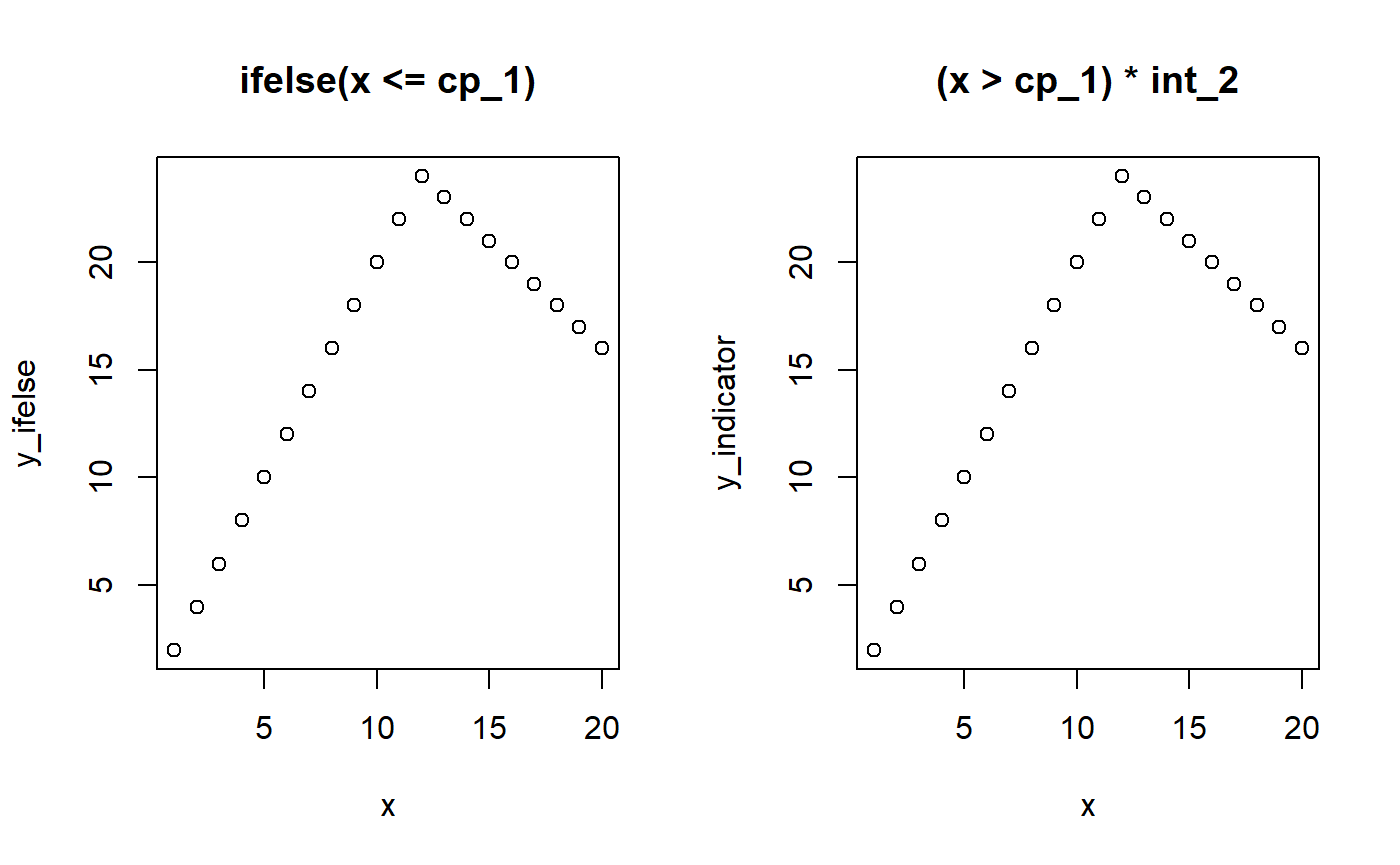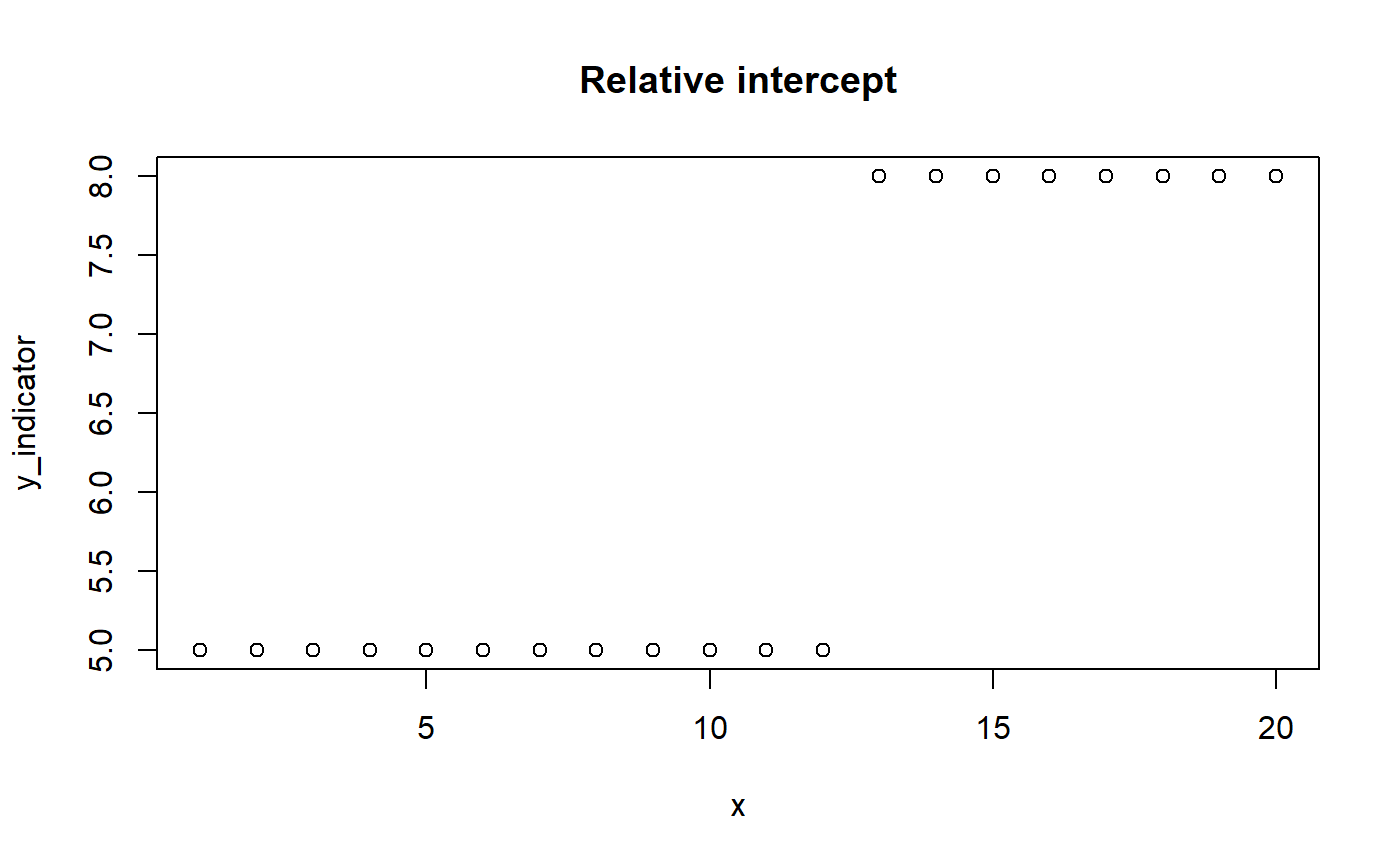mcp takes a list of formulas, and defines the change point as the point on the x-axis where the data shifts from being generated by one formula to the next. So with N formulas, you have N - 1 change points. A list with just one formula thus correspond to normal regression with 0 change points.

The formulas are called “segments” because they divide the (observed) x-axis into N segments.

# Formula format for segments

The format of each segment is generally response ~ changepoint ~ predictors + sigma(variance_predictors), except for the first segment where there is no change point (response ~ predictors + sigma(variance_predictors)). Here is a simple and a complex formula

• The response is just the name of a column in your data.
• The change point can be 1 for a population-level change point or 1 + (1|group) varying change points sampled around the population-level change point.
• The predictors can be 0 (no change in intercept), 1 (change in intercept), and any column in your data which you want to model a slope on.
• The variance predictors has it’s own article, but they follow the same rules as the predictors.

For convenience, you can omit the response and the change point in segment 2+, in which case the former response and an intercept-only (as opposed to random/varying) change point is assumed. When you call summary(fit), it will show the explicit representation. Let us see this in action for this model where we predict score as a function of time in three segments, i.e., with two change points:

library(mcp)
model = list(
score ~ 1,  # intercept
score ~ 1 ~ 0 + time,  # joined slope
~ time  # disjoined slope. "score ~ 1 ~ 1 + time" is implicit here.
)

# Interpret, but do not sample.
fit = mcp(model, sample = FALSE)
summary(fit)
## Family: gaussian(link = 'identity')
## Segments:
##   1: score ~ 1
##   2: score ~ 1 ~ 0 + time
##   3: score ~ 1 ~ time
##
## No samples. Nothing to summarise.

Notice how it added the response and the change point to the last segment?

mcp is heavily inspired by brms which again is inspired by lme4::lmer. Here is a bit of history on that.

# Parameter names

mcp automatically assigns names to the parameters in the format type_i where i is the segment number. Specifically:

• int_i is the intercept in the ith segment.
• year_i is the slope in on the data column year in the ith segment. x_i is the slope on the data column x in the ith segment. The slope takes name after the data it is regressed on.
• cp_i is the ith change point. Notice that cp_i is specified in segment i + 1. cp_1 occurs when there are two segments, and cp_2 when there are three segments, etc. OBS: future versions may start at cp_2..
• cp_i_group is the varying deviations from cp_i. See varying change points in mcp.
• cp_i_sd is the population-level standard deviation of the varying effects.
• sigma_* are variance parameters about which you can read more here). Note that for family = gaussian() and other families with an SD residual, there will always be an sigma_1, i.e., the common sigma initiated in the first segment.
• arj_i are autocorrelation coefficients of order j for segment i (read more here).

These parameter names are saved in fit$pars. Let us specify a somewhat complex model to show off some parameter names: model = list( # int_1 score ~ 1, # cp_1, cp_1_sd, cp_1_id, x_2 1 + (1|id) ~ 0 + year, # cp_2, cp_2_sd, cp_2_condition, int_2, x_2 1 + (1|condition) ~ 1 + rel(year) ) # Intepret, but do not sample. fit = mcp(model, sample = FALSE) str(fit$pars, vec.len = 99)  # Compact display
## List of 9
##  $x : chr "year" ##$ y         : chr "score"
##  $trials : NULL ##$ weights   : NULL
##  $varying : chr [1:2] "cp_1_id" "cp_2_condition" ##$ sigma     : chr "sigma_1"
##  $arma : chr(0) ##$ reg       : chr [1:8] "cp_1" "cp_1_sd" "cp_2" "cp_2_sd" "int_1" "int_3" "year_2" "year_3"
##  $population: chr [1:9] "cp_1" "cp_1_sd" "cp_2" "cp_2_sd" "int_1" "int_3" "year_2" "year_3" "sigma_1" ## - attr(*, "class")= chr [1:2] "mcplist" "list" # Modeling intercept change points A change point is simply like an ifelse statement or multiplying with indicators (0s and 1s): # Model parameters x = 1:20 cp_1 = 12 int_1 = 5 int_2 = 10 # Ifelse version y_ifelse = ifelse(x <= cp_1, yes = int_1, no = int_2) # Indicator equivalent using dummy helpers cp_0 = -Inf cp_2 = Inf y_indicator = (x > cp_0) * (x <= cp_1) * int_1 + # Between cp_0 and cp_1 (x > cp_1) * (x <= cp_2) * int_2 # Between cp_1 and cp_2 # Show it par(mfrow = c(1,2)) plot(x, y_ifelse, main = "ifelse(x <= cp_1)") plot(x, y_indicator, main = "(x > cp_1) * int_2")The magic of (Bayesian) MCMC sampling is that it can actually infer the change point from this simple formulation. We let mcp write the JAGS code for this simple two-plateaus model and see how it uses the indicator formulation of change points: model = list(y ~ 1, ~ 1) fit = mcp(model, sample = FALSE, par_x = "x") fit$jags_code
## model {
##
##   # Priors for population-level effects
##   cp_0 = MINX  # mcp helper value.
##   cp_2 = MAXX  # mcp helper value.
##
##   cp_1 ~ dunif(MINX, MAXX)
##   int_1 ~ dt(0, 1/(3*SDY)^2, 3)
##   int_2 ~ dt(0, 1/(3*SDY)^2, 3)
##   sigma_1 ~ dnorm(0, 1/(SDY)^2) T(0, )
##
##
##   # Model and likelihood
##   for (i_ in 1:length(x)) {
##     X_1_[i_] = min(x[i_], cp_1)
##     X_2_[i_] = min(x[i_], cp_2) - cp_1
##
##     # Fitted value
##     y_[i_] =
##
##       # Segment 1: y ~ 1
##       (x[i_] >= cp_0) * (x[i_] < cp_1) * int_1 +
##
##       # Segment 2: y ~ 1 ~ 1
##       (x[i_] >= cp_1) * int_2
##
##     # Fitted standard deviation
##     sigma_[i_] = max(0,
##       (x[i_] >= cp_0) * sigma_1 )
##
##     # Likelihood and log-density for family = gaussian()
##     y[i_] ~ dnorm((y_[i_]), 1 / sigma_[i_]^2)  # SD as precision
##     loglik_[i_] = logdensity.norm(y[i_], (y_[i_]), 1 / sigma_[i_]^2)  # SD as precision
##   }
## }

Look at the section called # Fitted value which is the (automatically generated) model that was discussed above. Some unnecessary stuff is added to segment 1 just because it makes the code easier to generate. (x[i_] >= cp_0 when cp_0 is the smallest value of x is, of course, always true).

# Modeling slope change points

We can use the same principle to model change points on slopes. However, we have to “take off” where the previous slope left us on the y-axis. That is, we have to regard whatever y-value the previous segment ended with as a kind of intercept-at-x=0 in the frame of the new segment. The intercept of segment 2 is cp_1 * slope_1 and the slope in segment 2 is x * (slope_2 - cp_1).

# Model parameters
x = 1:20
cp_1 = 12
slope_1 = 2
slope_2 = -1

# Ifelse version
y_ifelse = ifelse(x <= cp_1,
yes = slope_1 * x,
no = cp_1 * slope_1 + slope_2 * (x - cp_1))

# Indicator version. pmin() is a vectorized min()
cp_0 = -Inf
y_indicator = (x > cp_0) * slope_1 * pmin(x, cp_1) +
(x > cp_1) * slope_2 * (x - cp_1)

# Show it
par(mfrow = c(1,2))
plot(x, y_ifelse, main = "ifelse(x <= cp_1)")
plot(x, y_indicator, main = "(x > cp_1) * int_2")Let us see this in action:

model = list(y ~ 0 + x,
~ 0 + x)
fit = mcp(model, sample = FALSE)
fit$jags_code ## model { ## ## # Priors for population-level effects ## cp_0 = MINX # mcp helper value. ## cp_2 = MAXX # mcp helper value. ## ## cp_1 ~ dunif(MINX, MAXX) ## x_1 ~ dt(0, 1/(SDY/(MAXX-MINX))^2, 3) ## x_2 ~ dt(0, 1/(SDY/(MAXX-MINX))^2, 3) ## sigma_1 ~ dnorm(0, 1/(SDY)^2) T(0, ) ## ## ## # Model and likelihood ## for (i_ in 1:length(x)) { ## X_1_[i_] = min(x[i_], cp_1) ## X_2_[i_] = min(x[i_], cp_2) - cp_1 ## ## # Fitted value ## y_[i_] = ## ## # Segment 1: y ~ 0 + x ## (x[i_] >= cp_0) * x_1 * X_1_[i_] + ## ## # Segment 2: y ~ 1 ~ 0 + x ## (x[i_] >= cp_1) * x_2 * X_2_[i_] ## ## # Fitted standard deviation ## sigma_[i_] = max(0, ## (x[i_] >= cp_0) * sigma_1 ) ## ## # Likelihood and log-density for family = gaussian() ## y[i_] ~ dnorm((y_[i_]), 1 / sigma_[i_]^2) # SD as precision ## loglik_[i_] = logdensity.norm(y[i_], (y_[i_]), 1 / sigma_[i_]^2) # SD as precision ## } ## } Again, look at the #Fitted value to see the indicator-version in action. And again, mcp adds something about cp_0 = -Inf and cp_2 = Inf, just for internal convenience. You will find the exact same formula for y_ = ... if you do print(fit$simulate), though this function contains a whole lot of other stuff too.

# Modeling relative slopes and intercepts

mcp allows for specifying relative intercepts and change points through rel(). Relative slopes are easy: just replace x_2 with x_1 + x_2. You could do the same if all segments are intercept-only. However, if the previous segment had a slope, we want the intercept to be relative to where that “ended”. The mcp solution is to only “turn off” the “hanging intercept” from that slope’s ending (pmin(x, cp_i)) when the model encounters an absolute intercept. An indicator does this.

# Model parameters
x = 1:20
cp_1 = 12
int_1 = 5
int_2 = 3  # let's model this as relative

# Indicator version.
cp_0 = -Inf
y_indicator = (x > cp_0) * int_1 +  # Note: no (x < cp_1)
(x > cp_1) * (int_2)

# Plot it
plot(x, y_indicator, main = "Relative intercept")You can look at fit$jags_code and fit$simulate() to see this in action.